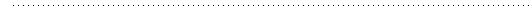...the official site for results from the National Assessment of Educational ProgressHome## Mathematics Sample QuestionsThree sample mathematics questions are presented in this section for each age. The response options for multiple-choice questions are provided as the students saw them, and the oval for the correct answer is filled in. All constructed-response questions in the long-term trend mathematics assessment were scored as correct or
incorrect, and the correct response is shown on the answer line.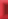Age 9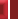Age 13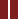Age 17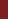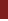Sample Question 1Sample Question 2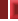Sample Question 3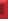Sample question 3 asked students to read a scale on a number line presented in the context of a bicycle speedometer. To answer the question, the student had to determine the value corresponding to a point halfway between the points marked at 10 and 20 miles per hour.

 85 percent of 9-year-olds answered this question correctly.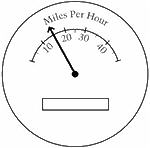The bicycle speedometer above shows about what speed?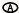10 miles per hour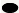15 miles per hour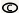20 miles per hour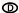45 miles per hour